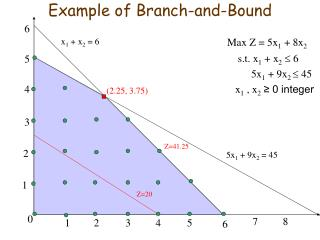Download PresentationExample of Branch-and-Bound

# Example of Branch-and-Bound - PowerPoint PPT PresentationDownload Presentation## Example of Branch-and-Bound

- - - - - - - - - - - - - - - - - - - - - - - - - - - E N D - - - - - - - - - - - - - - - - - - - - - - - - - - -
##### Presentation Transcript

1. 6 Example of Branch-and-Bound x1 + x2= 6 5 Max Z = 5x1 + 8x2 s.t. x1 + x2 6 5x1 + 9x2  45 x1 , x2≥ 0 integer 4 (2.25, 3.75) 3 Z=41.25 2 5x1 + 9x2= 45 1 Z=20 0 7 8 1 2 3 4 5 6

2. Branching step • Choose a variable that is fractional in the optimal solution to the LP-relaxation – say, x2 . Observe that every feasible IP point must have either x2  3 or x2 ≥ 4 . • With this in mind, branch on the variable x2to create the following two subproblems: Subproblem 1 Subproblem 2 Max Z = 5x1 + 8x2 Max Z = 5x1 + 8x2 s.t. x1 + x2 6 s.t. x1 + x2 6 5x1 + 9x2  45 5x1 + 9x2  45 x2  3 x2 ≥ 4 x1 , x2≥ 0 x1 , x2≥ 0 • Solve both subproblems (note that the original optimal solution (2.25, 3.75) can’t recur)

3. Branching step (graphically) Z=41 5 Subproblem 1: Opt. solution (3,3) with value 39 Subproblem 2: Opt. solution (1.8,4) with value 41 Subproblem 2 4 (1.8, 4) 3 (3, 3) 2 Subproblem 1 1 Z=39 Z=20 0 7 8 1 2 3 4 5 6

4. Solution tree S1: x2 3 (3, 3) Z=39 int. All (2.25, 3.75) Z=41.25 S2: x2≥ 4 (1.8, 4) Z=41 For each subproblem, we record • the restriction that creates the subproblem • the optimal LP solution • the LP optimum value The optimal solution for Subproblem 1 is integral: (3, 3). • If further branching on a subproblem will yield no useful information, then we can fathom (dismiss) the subproblem. In our case, we can fathom Subproblem 1 because its solution is integral. • The best integer solution found so far is stored as incumbent. The value of the incumbent is denoted by Z*. In our case, the first incumbent is (3, 3),and Z*=39. • Z* is a lower bound for OPT(IP): OPT(IP) ≥ Z* . In our case, OPT(IP) ≥ 39. The upper bound is 41: OPT(IP)  41.

5. Z=20 Next branching step (graphically) 5 (1, 4.44) Subpr. 4 - Fathom Subproblem 1. - Branch Subproblem 2 on x1 : Subproblem 3: New restriction is x1 1. Opt. solution (1, 4.44) with value 40.55 Subproblem 4: New restriction is x1≥ 2. The subproblem is infeasible Subpr. 3 4 3 Z=40.55 2 1 0 7 8 1 2 3 4 5 6

6. Solution tree (cont.) S1: x2 3 (3, 3) Z=39 int. All (2.25, 3.75) Z=41.25 S3: x1 1 (1, 4.44) Z=40.55 • If a subproblem is infeasible, then it is fathomed. In our case, Subproblem 4 is infeasible; fathom it. • The upper bound for OPT(IP) is updated: 39OPT(IP)  40.55 . • Next branch Subproblem 3 on x2 . (Note that the branching variable might recur). S2: x2≥ 4 (1.8, 4) Z=41 S4: x1≥ 2 infeasible

7. Z=20 Next branching step (graphically) (0, 5) 5 Branch Subproblem 3 on x2 : Subproblem 5: New restriction is x2 4. Feasible region: the segment joining (0,4) and (1,4) Opt. solution (1, 4) with value 37 Subproblem 6: New restriction is x2≥ 5. Feasible region is just one point: (0, 5) Opt. solution (0, 5) with value 40 4 (1, 4) 3 2 1 0 7 8 1 2 3 4 5 6

8. Solution tree (final) S1: x2 3 (3, 3) Z=39 int. S5: x2 4 (1, 4) Z=37 int. All (2.25, 3.75) Z=41.25 S3: x1 1 (1, 4.44) Z=40.55 • If the optimal value of a subproblem is  Z*, then it is fathomed. • In our case, Subproblem 5 is fathomed because 37  39 = Z*. • If a subproblem has integral optimal solution x*, and its value > Z*, then x* replaces the current incumbent. • In our case, Subproblem 5 has integral optimal solution, and its value 40>39=Z*. Thus, (0,5) is the new incumbent, and new Z*=40. • If there are no unfathomed subproblems left, then the current incumbent is an optimal solution for (IP). • In our case, (0, 5) is an optimal solution with optimal value 40. S2: x2≥ 4 (1.8, 4) Z=41 S6: x2≥ 5 (0, 5) Z=40 int. S4: x1≥ 2 infeasible